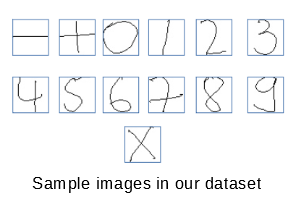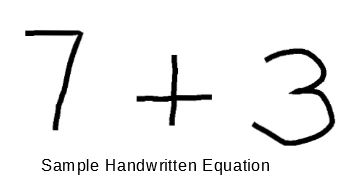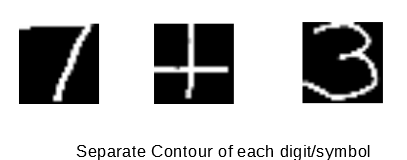# Handwritten Equation Solver in Python

• Difficulty Level : Medium
• Last Updated : 26 Jun, 2019

Acquiring Training Data

Download the dataset from this link. Extract the zip file. There will be different folders containing images for different maths symbol. For simplicity, use 0–9 digits, +, ?-?and, times images in our equation solver. On observing the dataset, we can see that it is biased for some of the digits/symbols, as it contains 12000 images for some symbol and 3000 images for others. To remove this bias, reduce the number of images in each folder to approx. 4000.
• Extracting Features
We can use contour extraction to obtain features.

1. Invert the image and then convert it to a binary image because contour extraction gives the best result when the object is white, and surrounding is black.
2. To find contours use ‘findContour’ function. For features, obtain the bounding rectangle of contour using ‘boundingRect’ function (Bounding rectangle is the smallest horizontal rectangle enclosing the entire contour).
3. Since each image in our dataset contains only one symbol/digit, we only need the bounding rectangle of maximum size. For this purpose, we calculate the area of the bounding rectangle of each contour and select the rectangle with maximum area.
4. Now, resize the maximum area bounding rectangle to 28 by 28. Reshape it to 784 by 1. So there will be now 784-pixel values or features. Now, give the corresponding label to it (For e.g., for 0–9 images same label as their digit, for – assign label 10, for + assign label 11, for times assign label 12). So now our dataset contains 784 features column and one label column. After extracting features, save the data to a CSV file.
•Training Data using Convolutional Neural Network

Since convolutional neural network works on two-dimensional data and our dataset is in the form of 785 by 1. Therefore, we need to reshape it. Firstly, assign the labels column in our dataset to variable y_train. Then drop the labels column from the dataset and then reshape the dataset to 28 by 28. Now, our dataset is ready for CNN.
• Building Convolutional Neural Network
For making CNN, import all the necessary libraries.

 `import` `pandas as pd``import` `numpy as np``import` `pickle``np.random.seed(``1212``)``import` `keras``from` `keras.models ``import` `Model``from` `keras.layers ``import` `*` `from` `keras ``import` `optimizers``from` `keras.layers ``import` `Input``, Dense``from` `keras.models ``import` `Sequential``from` `keras.layers ``import` `Dense``from` `keras.layers ``import` `Dropout``from` `keras.layers ``import` `Flatten``from` `keras.layers.convolutional ``import` `Conv2D``from` `keras.layers.convolutional ``import` `MaxPooling2D``from` `keras.utils ``import` `np_utils``from` `keras ``import` `backend as K``K.set_image_dim_ordering(``'th'``)``from` `keras.utils.np_utils ``import` `to_categorical``from` `keras.models ``import` `model_from_json`

Convert the y_train data to categorical data using ‘to_categorical’ function. For making model, use the following line of code.

 `model ``=` `Sequential()``model.add(Conv2D(``30``, (``5``, ``5``), input_shape ``=``(``1``, ``28``, ``28``), activation ``=``'relu'``))``model.add(MaxPooling2D(pool_size ``=``(``2``, ``2``)))``model.add(Conv2D(``15``, (``3``, ``3``), activation ``=``'relu'``))``model.add(MaxPooling2D(pool_size ``=``(``2``, ``2``)))``model.add(Dropout(``0.2``))``model.add(Flatten())``model.add(Dense(``128``, activation ``=``'relu'``))``model.add(Dense(``50``, activation ``=``'relu'``))``model.add(Dense(``13``, activation ``=``'softmax'``))``# Compile model``model.``compile``(loss ``=``'categorical_crossentropy'``, ``              ``optimizer ``=``'adam'``, metrics ``=``[``'accuracy'``])`

• Fitting Model to Data
For fitting CNN to data use the following lines of code.

 `model.fit(np.array(l), cat, epochs ``=` `10``, batch_size ``=` `200``, ``          ``shuffle ``=` `True``, verbose ``=` `1``)`

It will take around three hours to train our model with an accuracy of 98.46%. After training, we can save our model as json file for future use, So that we don’t have to train our model and wait for three hours every time. To save our model, we can use the following line of codes.

 `model_json ``=` `model.to_json()``with ``open``(``"model_final.json"``, ``"w"``) as json_file:``    ``json_file.write(model_json)``# serialize weights to HDF5``model.save_weights(``"model_final.h5"``)`

• Testing our Model or Solving Equation using it

Firstly, import our saved model using the following line of codes.

 `json_file ``=` `open``(``'model_final.json'``, ``'r'``)``loaded_model_json ``=` `json_file.read()``json_file.close()``loaded_model ``=` `model_from_json(loaded_model_json)``# load weights into new model``loaded_model.load_weights(``"model_final.h5"``)`

• Now, input an image containing a handwritten equation. Convert the image to a binary image and then invert the image(if digits/symbols are in black).
• Now obtain contours of the image, by default, it will obtain contours from left to right.
• Obtain bounding rectangle for each contour.
• Sometimes, it will result in two or more contours for the same digit/symbol. To avoid that, check if the bounding rectangle of those two contours overlaps or not. If they overlap, then discard the smaller rectangle.
• Now, resize all the remaining bounding rectangle to 28 by 28.
• Using the model, predict the corresponding digit/symbol for each bounding rectangle and store it in a string.
• After that use ‘eval’ function on the string to solve the equation.
•